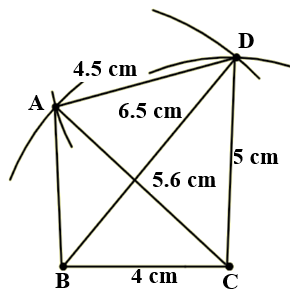Construct a quadrilateral ABCD in which BC = 4 cm, CA = 5.6 cm, AD = 4.5 cm, CD = 5 cm and BD = 6.5 cm.

Asked by Aaryan | 1 year ago |  52

##### Solution :-

The given details are BC = 4 cm, CA = 5.6 cm, AD = 4.5 cm, CD = 5 cm and BD = 6.5 cm.

Step 1- Draw a line BC = 4cm

Step 2- Cut an arc of radius 6.5cm with B as the center to mark that point as D.

Step 3- Cut an arc of radius 5cm with C as the center to intersect at point D.

Step 4- Cut an arc of radius 5.6cm with C as the center to mark that point as A.

Step 5- Cut an arc of radius 4.5cm with D as the center to intersect at point A.

Step 6- Now join BD, CD, CA, DA and ABAnswered by Sakshi | 1 year ago

### Related Questions

#### Construct a quadrilateral PQRS in which PQ = 4 cm, QR = 5 cm ∠P = 50°, ∠Q = 110° and ∠R = 70°.

Construct a quadrilateral PQRS in which PQ = 4 cm, QR = 5 cm ∠P = 50°, ∠Q = 110° and ∠R = 70°.

#### Construct a quadrilateral ABCD ∠A = 65°, ∠B = 105°, ∠C = 75°, BC = 5.7 cm and CD = 6.8 cm.

Construct a quadrilateral ABCD ∠A = 65°, ∠B = 105°, ∠C = 75°, BC = 5.7 cm and CD = 6.8 cm.

#### Construct a quadrilateral ABCD when BC = 5.5 cm, CD = 4.1 cm, ∠A = 70°, ∠B = 110° and ∠D = 85°.

Construct a quadrilateral ABCD when BC = 5.5 cm, CD = 4.1 cm, ∠A = 70°, ∠B = 110° and ∠D = 85°.